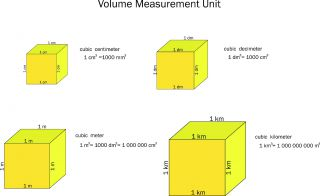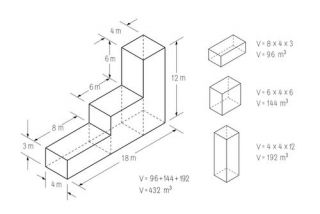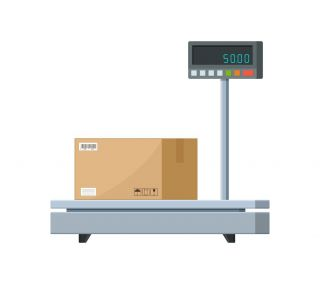# What Is CBM and How Do You Calculate It?

JANUARY 28, 2021 BY RYAN STOLTZ

• SHARE ARTICLE:CBM is one of the most frequently used terms in air, sea, and road transportation. If you've ever bought, rented, or read about shipping containers, you may also have noticed the term mentioned in the container specifications. So, what exactly is CBM, and why is it important?

Calculating the CBM helps determine the road, air, and sea freight charges for transporting goods over large distances. Similarly, if you're using a shipping container, CBM shows the amount of storage that you'll get.

CBM stands for Cubic Meter. It denotes the volumetric capacity of a shipping container or cargo unit. Below, we’ll take a closer look into CMB, how it is calculated, and how transportation companies arrive at freight charges based on the measurement.

## What Is CBM?

Cubic meter (CBM) is the unit of measuring the shipment volume. It denotes the available space for your shipping goods. The larger the CBM, the higher the volume of goods in the shipment. The more goods you ship, the higher the charges become.

You can check out the 20ft container and 40ft container dimensions on our blog.### Why Does Measuring in Cubic Meters Matter?

There are two ways you can transport goods over large distances - full container load (FCL) and less container load (LCL). If you're transporting a full container load, you have to pay charges on an FCL basis.

On the contrary, for LCL shipments, a freight forwarder will levy charges based on the weight calculated using the cargo volume. Therefore, calculating the CBM can provide some good insight into the transportation charges.

While ocean freight generally moves only through commercial vessels, there are multiple ways of moving shipping containers on the road. Calculating the CBM for road transport can help you select the most appropriate means of transportation, such as trailers, trucks, flat-rack vehicles, etc.

## How to Calculate CBM

The process of calculating the CBM of a shipment is pretty straightforward.

Multiply the length, width, height, and number of items to get the CBM.

We can write the formula for cubic meter capacity as:

Total CBM = Length x Width x Height x Number of Items

Let's walk through an example. Suppose you have ten boxes of 2 m length, 1 m height, and 1 m width, and you need to find the freight volume or the total shipment volume. In this case, the steps for finding the total volume or CBM will be:

CBM = Length x Width x Height = 2 x 1 x 1 = 4 m3
Number of boxes = 10

Therefore, total CBM = CBM x Number of Items = 4 x 10 = 40 m3

You can find several online tools to calculate the CBM. However, even the most sophisticated CBM calculator multiplies the length, width, and height of the cargo.

There are certain exceptions to this formula. For instance, if your boxes are not identical, you need to calculate the individual volume using the CBM formula. Then you must add these volumes to find the total storage capacity in cubic meters.

Suppose you have three boxes of 4 m3, 8 m3, and 10 m3 CBM each. In this case the total volume = 4 + 8 + 10 = 22 m3.In every CBM calculation, we assume that the cartons are rectangular with a certain height. Therefore, if you have irregular-shaped cargo, you must modify the formula to calculate CBM.

### What Metrics are Required for Calculation?

Every CBM calculator requires the length, width, height, and a number of items. However, it is critical to note that all the dimensions must be in the same unit.

If you measure the dimensions of the cargo in inches, you'll get the volume in cubic inches. You'll then have to convert the cubic inches into cubic meters. Similarly, you can also calculate the volume in cubic feet but ensure that you've taken the height, width, and length in feet.

For heavier items, gross weight is more important than CBM because the freight charges will depend on weight instead. With that, the next important step in any freight calculation is determining the weight of the cargo.

## What's the Difference Between Volumetric Weight and Chargeable Weight?

There are several reasons why we calculate both the volume and the weight of the shipment. Although it is assumed the transportation cost of the cargo depends upon its weight, it is not true in all cases.

Let's say you're transporting large Thermocol sheets or styrofoam cups. In such cases, the weight is quite negligible compared to the volume that your shipment will occupy. Alternatively, if you're transporting small steel parts, the shipment will take up less space, but it will be heavy.

If we levy charges based on the CBM, steel parts will have a very low rate despite their significant weight. On the contrary, when we calculate the freight cost based on the weight, lighter items like cotton and plastics will incur low international freight charges.

When it comes to freight calculations, the actual weight of the cargo and the space that it occupies are equally important. Therefore, we use the concept of dimensional or volumetric weight and the chargeable weight.

Let's explore how we arrive at the concept of volumetric and chargeable weight using the following terms:

### Gross Weight

Gross weight refers to the actual weight of your shipment, including the packaging, support, and storage pallets. Say you have five boxes, weighing 10 kg (22.04 lbs.) each plus 5 kg (11.02 lbs.) of cushioning and packaging materials. The gross weight will be 5 x 10 + 5 = 55 kg (121.25 lbs.)### Dimensional or Volumetric Weight

When we convert the total CBM of the cargo into equivalent weight using a multiplying factor known as the DIM factor, otherwise known as dimensional weight. Hence, the formula for Volumetric/Dimensional weight is Volumetric Weight = CBM x DIM Factor. An alternative formula that you can use is Dimensional Weight = Length (cm) x Width (cm) x Height (cm)/DIM Factor.

### What is the DIM Factor?

The Dimensional Weight Factor or DIM is a multiplication factor that depends upon the mode of transport. For instance, the DIM factor for sea cargo is different from the air freight factor. The DIM factor is a standard quantity based on various shipment types that helps freight companies determine their transport rate. The typical DIM factors are as follows:

Sea: 1:1000
Air: 1:6000
Courier/Express Freight: 1:5000

Say, for instance, you're transporting 1 CBM or 1 m3 of shipment through the sea. In this case, the dimensional weight of the cargo will be 1/(1:1000)=1000 kg.

While we've used standard DIM factors, their values may change according to local, domestic and international freight guidelines. Some companies may also use their own factors.

### Chargeable Weight

The weight that the company uses for levying freight charges is known as the chargeable weight. A freight forwarder will calculate the dimensional weight and the gross weight of your shipment. Instead of randomly selecting either of the two, your freight service provider will choose whichever is greater.

Consider you're shipping bulky but light shipments with a volumetric weight of 1000 kgs due to higher CBM while their gross weight is 500 kgs. The freight company will charge you based on the dimensional weight, i.e., 1000 kgs.

## How to Calculate Freight Using CBM

Once we know the freight rates, the gross weight, and the CBM, we can calculate the transportation charges for most common-sized shipping containers through the sea, air, or land. Let's explore how we arrive at the freight rate for the different means of transport after we calculate CBM.

### 1. Ocean

A less than container load (LCL) shipment depends on the specific DIM factor for sea, the freight rate per ton, and the CBM. We can then calculate the LCL ocean freight pricing using the following formula:

Total Freight Charges = Freight Charges per Ton (1000 kg) x Chargeable Weight in Ton (1000 kg)

Suppose you're sending a package of 2 m x 2 m x 2 m with a gross weight of 5000 kg. The company levies a charge of \$100/ton.

• Here, CBM = Length x Width x Height = 2 x 2 x 2 = 8 m3
• Dimensional Weight (DW) = CBM x DIM Factor for Sea = 8 x 1000 = 8,000 kg = 8 ton
• Gross weight (GW) = 5000 kg = 5 ton
• Since DW is greater than the GW, the freight company will consider the dimensional weight as the chargeable weight.
• Now, Total freight charges = Freight Charges per Ton (1000 kg) x Chargeable Weight in Ton (1000 kg) = \$100 x 8 = \$800

Consider that the GW of your shipment was 9000 kg (9 tons). As the GW is more significant than the DW, the freight provider considers GW the chargeable weight.

Now, Total freight charges = Freight Charges per Ton (1000 kg) x Chargeable Weight in Ton (1000 kg) = \$100 x 9 = \$900.

### 2. Air

The calculation steps remain the same for air freight, the only difference being the change in the dimensional factor. Let's calculate the freight charges for air cargo.

Say you're transporting a box measuring 200 cm x 100 cm x 60 cm with a gross weight of 100 kg. The company levies a freight rate of \$100/ton.

Note that since the dimensions are in cm, we need to use the formula -  Dimensional Weight = Length (cm) x Width (cm) x Height (cm)/DIM Factor to find the actual capacity.

• Here, CBM = Length x Width x Height = 200 x 100 x 60 = 1,200,000 cm3
• Dimensional Weight (DW) = CBM / DIM Factor for Air =  1,200,000/ 6000= 200 kgs = 0.2 ton
• Gross Weight (GW) = 100 kg = 0.1 ton
• Since DW is greater than the GW, the freight company will consider the dimensional weight as the chargeable weight.
• Now, Total freight charges = Freight Charges per ton x Chargeable Weight in ton = \$100 x 0.2 = \$20

Most trucks charge you based on the container's maximum capacity to transport a full load container. However, we're considering an example of less than load (LTL) transport, i.e., a partially filled container or a high-cube. Most LTL trucking carriers will charge according to the following calculations.

Let's say you're transporting a 300 cm x 100 cm x 200  cm carton with an actual weight of 500 kg. The road transport company agrees to a \$150/ton freight rate. Just like the air freight example, the dimensions are in cm. As such, we need to use the formula:  Dimensional Weight = Length (cm) x Width (cm) x Height (cm)/DIM Factor to find the actual capacity.

• Here, CBM = Length x Width x Height = 100 x 30 x 20 = 60,000 cm3
• Dimensional Weight (DW) = CBM / DIM Factor for Air =  6,000,000/ 6000= 1000 kgs = 1 ton
• Gross weight (GW) = 500 kg = 0.5 ton
• Since DW is more than the GW, the freight company will consider the dimensional weight as the chargeable weight.
• Now, Total freight charges = Freight Charges per ton x Chargeable Weight in ton = \$150 x 1 = \$150Please note that these calculations are indicative and do not represent actual figures.

So far, we've only discussed these measurements in reference to a rectangular prism. But, how do you calculate the CBM of a cylindrical package or an irregular object? Read on below to find out.

## How to Calculate the CBM of Irregularly Shaped Packages

We can calculate the cubic meter volume of any shape using standard formulae, as shown in the figure given below. If you have a cylindrical box, the formula to calculate CBM is π x r x r x h where 'r' is the radius of the cylinder, 'h' is its height, and π (pi) is a constant approximately equal to 3.14.

Suppose your objects are highly irregular that do not conform to any standard shape. In that case, CBM = length of the largest side x largest width x largest height.

## Impact of CBM on Freight Rates

While CBM is vital for calculating the freight charges, it also plays a crucial role in determining the additional charges and surcharges. Most companies levy the following amounts based on the CBM:

• Terminal Handling Charge: This refers to the cost of using the facilities, equipment, and labor at the origin and destination terminals.
• Internal Haulage Charges: These are the expenses incurred while moving the cargo from the container depot or the freight station to the loading port and vice versa.
• Bunker Adjustment Factor/Fuel Adjustment Factor: These are the surcharges to account for fuel price fluctuations
• Currency Adjustment Factor: This is an additional charge that companies levy for international freights to account for currency rate fluctuations to the base currency exchange.## Conclusion

Calculating the cubic meter capacity (CBM) of your shipment is essential for determining the freight charges. As the freight rates can vary significantly according to the means of transportation, knowing how to calculate CBM can help you create an efficient logistics plan. CBM also helps you understand the total weight of your cargo and select the most appropriate means of transport.## FAQs

### What Is the Formula to Calculate CBM?

We can use the following formula to calculate CBM:
Total CBM = Length of each item x Width of each item x Height of each item x Number of Items

### How Many CBM is 1 kg?

We can calculate the CBM in kgs by multiplying the total freight volume with the dimensional factor, such that,

Volumetric Weight = CBM x DIM Factor

The dimensional factor varies for sea, air, and road transport. A 60 cm x 10 cm x 10 cm (6000 CBM) box used in air transport (DIM - 1:6000) is 1 kg (volumetric weight). Similarly, a 10 cm x 10 cm x 10 cm carton (1000 CBM) in ocean freight is 1 kg (volumetric weight).

### How Do You Calculate CBM in cm.?

First, you must measure the length, width, and height of your shipment in centimeters (cm). Convert these dimensions into meters by dividing them by 100 (1 cm = 1/100 m). Once you get the values in meters, use the CBM formula to calculate the cubic capacity.